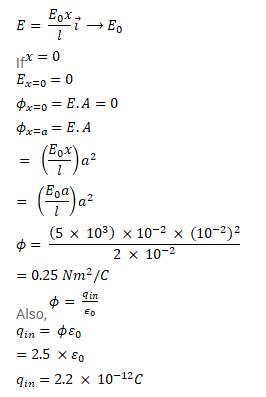# The electric field in a region is given byQuestion:

The electric field in a region is given by $\vec{E}=\frac{E_{0} x}{l} i$.

Find the charge contained inside a cubical volume bounded by the surfaces $x=0, x=a, y=a, z=0$ and $z=a$. Take $E_{0}=5 \times 10^{3} \mathrm{~N} / \mathrm{c}, l=2 \mathrm{~cm}$ and $a=1 \mathrm{~cm}$

Solution: